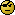## Hey, python is not so difficult :p [Power / Sample Size]

Hi BE-proff,

Syntatically it is not so hard, I think.

Below are some functions that will get you started. They execute just fine as a script on my computer (Win10). The functions use Simpson integrals and various constants that that you can play around with to achieve the combination of accuracy and speed that suits you. Note that I only made step 1-3, so I will leave it to you to put these into Potvin's equation.
They are in no way optimized so there's plenty of work to do stillimport math def GammaB(z):  x=0.0  dx=0.04  integral=0.0  di=10  it=0  while ((di>0.001) or  (x<8*z)):   y =pow(x, z-1)*math.exp(-x)   y1=pow(x+dx, z-1)*math.exp(-(x+dx))   y2=pow(x+dx+dx, z-1)*math.exp(-(x+dx+dx))   di= (dx/3)*(y+4*y1+y2)   integral=integral +di   x=x+dx+dx   it+=1  return(integral) def DensityB(x, df):  a=GammaB((df+1)/2.0 )  b=math.sqrt(df*math.pi) * GammaB(df/2.0 )  c=math.pow(1.0+x*x/df, -( (df+1) / 2.0))  return(a*c/b) def probtcum(df, t): ## note: for t>0 only, you can easily fix it for negative t  x=0.0  integral=0.5  dx=0.5*t/100  i=0  while (i<100):   y=DensityB(x, df)   y1=DensityB(x+dx, df)   y2=DensityB(x+dx+dx, df)    di = (dx/3)* (y+4*y1+y2)   integral=integral+di   x=x+dx+dx   i+=1        return(integral) def critvalt(df, p): ##fix it yourself for p lowe than 0.5x=0.0  integral=0.5  dx=0.004  while (integral<p):   y=DensityB(x, df)   y1=DensityB(x+dx, df)   y2=DensityB(x+dx+dx, df)    di = (dx/3)* (y+4*y1+y2)   integral=integral+di   x=x+dx+dx  ##aha!! now the solution is between x and x-2dx  ##so we can just interpolate linearly  a=di / (dx+dx)  b=integral-a*x  soln=(p-b) /a  return(soln) ## in R, pt(df=5, 0.4) is 0.6471634 p=probtcum(5, 0.4) print("probt cumul at df=5 for x=0.4", p, "should be", 0.6471634) ## in R, qt(df=11, 0.95) is 1.795885 q=critvalt(11, 0.95) print("critt at df=11 and p=0.95=", q, "should be", 1.795885) 

On my machine I get:

 >>> RESTART: [blahblah] probt cumul at df=5 for x=0.4 0.6471629404086429 should be 0.6471634 critt at df=11 and p=0.95= 1.7958969293454883 should be 1.795885 >>>Pass or fail!
ElMaestroIng. Helmut Schütz